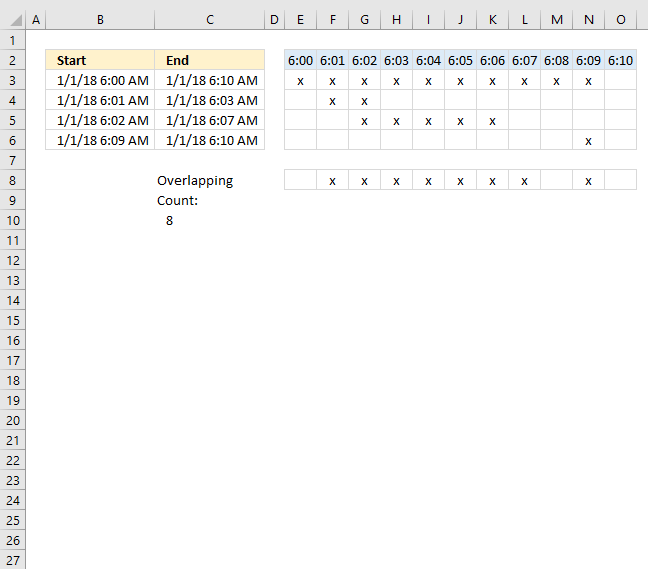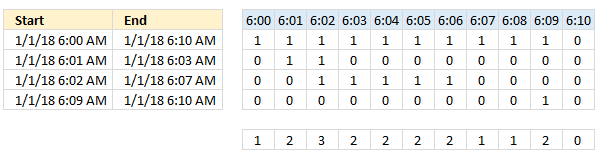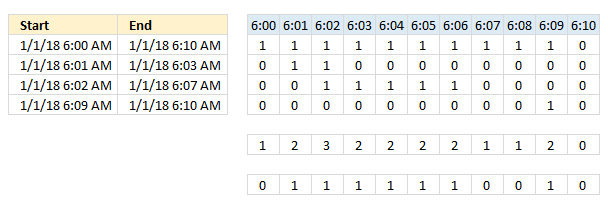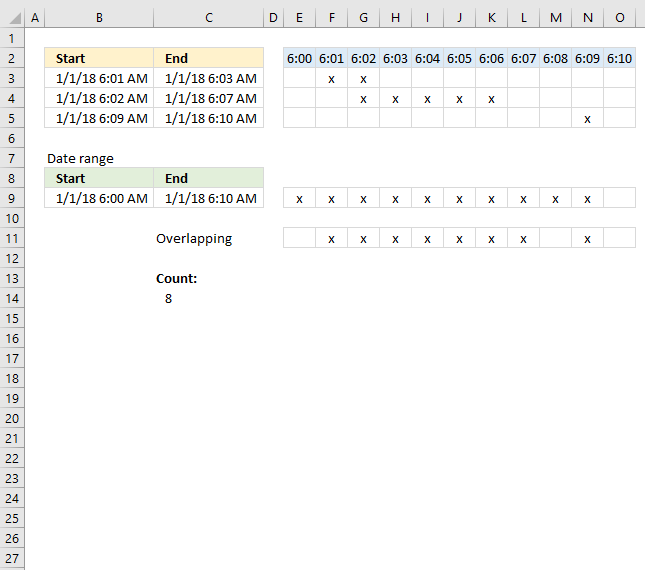Author: Oscar Cronquist Article last updated on October 09, 2018The worksheet above shows four different time ranges in column B and C, the formula in cell C10 counts the number of overlapping minutes.

The formula uses the earliest and latest date and time value in column B and C as the range to count overlapping minutes.

Array formula in cell C10:

=SUM((MMULT(TRANSPOSE(\$B\$3:\$B\$6^0), (TRANSPOSE(MIN(\$B\$3:\$B\$6)+(ROW(A1:INDEX(\$A:\$A, (MAX(\$C\$3:\$C\$6)-MIN(\$B\$3:\$B\$6))*1440+1))-1)/1440)>=\$B\$3:\$B\$6)*((TRANSPOSE(MIN(\$B\$3:\$B\$6)+(ROW(A1:INDEX(\$A:\$A, (MAX(\$C\$3:\$C\$6)-MIN(\$B\$3:\$B\$6))*1440+1))-1)/1440))<\$C\$3:\$C\$6))>1)*1)

To enter an array formula, type the formula in a cell then press and hold CTRL + SHIFT simultaneously, now press Enter once. Release all keys.

The formula bar now shows the formula with a beginning and ending curly bracket telling you that you entered the formula successfully. Don't enter the curly brackets yourself.

### Explaining formula in cell C10

The INDEX function allows you to create an array of values, in this case, minute intervals.

MIN(\$B\$3:\$B\$6)+(ROW(A1:INDEX(\$A:\$A, (MAX(\$C\$3:\$C\$6)-MIN(\$B\$3:\$B\$6))*1440+1))-1)/1440

returns

{43101.25, 43101.2506944444, 43101.2513888889, 43101.2520833333, 43101.2527777778, 43101.2534722222, 43101.2541666667, 43101.2548611111, 43101.2555555556, 43101.25625, 43101.2569444444}

Now check if these minute intervals are between or equal to each date and time range.

(TRANSPOSE(MIN(\$B\$3:\$B\$6)+(ROW(A1:INDEX(\$A:\$A, (MAX(\$C\$3:\$C\$6)-MIN(\$B\$3:\$B\$6))*1440+1))-1)/1440)>=\$B\$3:\$B\$6)*((TRANSPOSE(MIN(\$B\$3:\$B\$6)+(ROW(A1:INDEX(\$A:\$A, (MAX(\$C\$3:\$C\$6)-MIN(\$B\$3:\$B\$6))*1440+1))-1)/1440))<\$C\$3:\$C\$6)

returns

{1, 1, 1, 1, 1, 1, 1, 1, 1, 1, 0; 0, 1, 1, 1, 0, 0, 0, 0, 0, 0, 0; 0, 0, 1, 1, 1, 1, 1, 1, 0, 0, 0; 0, 0, 0, 0, 0, 0, 0, 0, 0, 1, 0}

The MMULT function allows you to add these values column by column.

MMULT(TRANSPOSE(\$B\$3:\$B\$6^0), (TRANSPOSE(MIN(\$B\$3:\$B\$6)+(ROW(A1:INDEX(\$A:\$A, (MAX(\$C\$3:\$C\$6)-MIN(\$B\$3:\$B\$6))*1440+1))-1)/1440)>=\$B\$3:\$B\$6)*((TRANSPOSE(MIN(\$B\$3:\$B\$6)+(ROW(A1:INDEX(\$A:\$A, (MAX(\$C\$3:\$C\$6)-MIN(\$B\$3:\$B\$6))*1440+1))-1)/1440))<\$C\$3:\$C\$6))

becomes

MMULT(TRANSPOSE(\$B\$3:\$B\$6^0), {1, 1, 1, 1, 1, 1, 1, 1, 1, 1, 0; 0, 1, 1, 1, 0, 0, 0, 0, 0, 0, 0; 0, 0, 1, 1, 1, 1, 1, 1, 0, 0, 0; 0, 0, 0, 0, 0, 0, 0, 0, 0, 1, 0})

becomes

MMULT({1,1,1,1}, {1, 1, 1, 1, 1, 1, 1, 1, 1, 1, 0; 0, 1, 1, 1, 0, 0, 0, 0, 0, 0, 0; 0, 0, 1, 1, 1, 1, 1, 1, 0, 0, 0; 0, 0, 0, 0, 0, 0, 0, 0, 0, 1, 0})

and returns

{1, 2, 3, 3, 2, 2, 2, 2, 1, 2, 0}

The following picture shows the array and what the MMULT function returns.A value larger than 1 indicates an overlapping time value.

MMULT(TRANSPOSE(\$B\$3:\$B\$6^0), (TRANSPOSE(MIN(\$B\$3:\$B\$6)+(ROW(A1:INDEX(\$A:\$A, (MAX(\$C\$3:\$C\$6)-MIN(\$B\$3:\$B\$6))*1440+1))-1)/1440)>=\$B\$3:\$B\$6)*((TRANSPOSE(MIN(\$B\$3:\$B\$6)+(ROW(A1:INDEX(\$A:\$A, (MAX(\$C\$3:\$C\$6)-MIN(\$B\$3:\$B\$6))*1440+1))-1)/1440))<=\$C\$3:\$C\$6))>1

becomes

{1, 2, 3, 3, 2, 2, 2, 2, 1, 2, 0}>1

and returns

{FALSE, TRUE, TRUE, TRUE, TRUE, TRUE, TRUE, TRUE, FALSE, TRUE, FALSE}.

Lastly, multiply with 1 to convert boolean values to numerical values and then sum the numbers.

SUM((MMULT(TRANSPOSE(\$B\$3:\$B\$6^0), (TRANSPOSE(MIN(\$B\$3:\$B\$6)+(ROW(A1:INDEX(\$A:\$A, (MAX(\$C\$3:\$C\$6)-MIN(\$B\$3:\$B\$6))*1440+1))-1)/1440)>=\$B\$3:\$B\$6)*((TRANSPOSE(MIN(\$B\$3:\$B\$6)+(ROW(A1:INDEX(\$A:\$A, (MAX(\$C\$3:\$C\$6)-MIN(\$B\$3:\$B\$6))*1440+1))-1)/1440))<=\$C\$3:\$C\$6))>1)*1)

becomes

SUM({FALSE, TRUE, TRUE, TRUE, TRUE, TRUE, TRUE, TRUE, FALSE, TRUE, FALSE}*1)

becomes

SUM({0,1,1,1,1,1,1,1,0,1,0})and returns 8.The picture above shows you a different setup, it allows you to use a smaller range than the min and max date and time in B3:C5.

Formula in cell C14:

=SUM((MMULT(TRANSPOSE(\$B\$3:\$B\$5^0), (TRANSPOSE(\$B\$9+(ROW(A1:INDEX(\$A:\$A, (\$C\$9-\$B\$9)*1440+1))-1)/1440)>=\$B\$3:\$B\$5)*((TRANSPOSE(\$B\$9+(ROW(A1:INDEX(\$A:\$A, (\$C\$9-\$B\$9)*1440+1))-1)/1440))<\$C\$3:\$C\$5))>0)*1)

The ROW function limits the use of these formulas, if you have a range larger than 1,048,576 minutes, which is the same as the number of rows in a worksheet, you will need another solution than the one presented here.